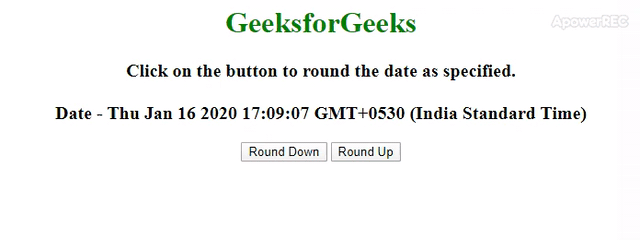# How to Round off Time to Nearest 5 Min using JavaScript ?

Given a JavaScript date and the task is to round it to 5 minutes with the help of JavaScript. There are two approaches that are discussed below:

Approach 1: In this approach, both options are available to either round down or round up the date object. This example uses basic Math.floor() function and Math.ceil() function to perform the operation.

• Example: This example implements the above approach.

 ` ` `<``html``> ` ` `  `<``head``> ` `    ``<``title``> ` `        ``Round off a Date Object to 5 ` `        ``minutes in JavaScript. ` `    `` ` ` ` ` `  `<``body` `style``=``"text-align:center;"``> ` `     `  `    ``<``h1` `style``=``"color:green;"``> ` `        ``GeeksforGeeks ` `    `` ` `     `  `    ``<``p` `id``=``"gfg"` `style="font-size: 20px;  ` `                    ``font-weight: bold"> ` `    `` ` `     `  `    ``<``button` `onclick``=``"GFG_Fun1();"``> ` `        ``Round Down ` `    `` ` `     `  `    ``<``button` `onclick``=``"GFG_Fun2();"``> ` `        ``Round Up ` `    `` ` `     `  `    ``<``p` `id``=``"geeks"` `style="font-size: 26px;  ` `            ``font-weight: bold;color: green;"> ` `    `` ` `     `  `    ``<``script``> ` `        ``var up = document.getElementById('gfg'); ` `        ``var down = document.getElementById('geeks'); ` `        ``var date = new Date(); ` `        ``up.innerHTML = "Click on the button to " ` `                ``+ "round the date as specified." ` `                ``+ "<``br``><``br``>Date - " + date; ` ` `  `        ``function GFG_Fun1() { ` `             `  `            ``// ms in 5 minutes. ` `            ``var coff = 1000 * 60 * 5;  ` `             `  `            ``down.innerHTML = new Date( ` `                    ``Math.floor(date / coff) * coff); ` `        ``} ` ` `  `        ``function GFG_Fun2() { ` `             `  `            ``// ms in 5 minutes. ` `            ``var coff = 1000 * 60 * 5;  ` `            ``down.innerHTML = new Date( ` `                    ``Math.ceil(date / coff) * coff); ` `        ``} ` `    `` ` ` ` ` `  ` `

• Output:Approach 2: This example uses basic Math.round() function to perform the operation. Calculate the milliseconds in 5 minutes, divide the date object by milliseconds and get the round value then again multiply the milliseconds.

• Example: This example implements the above approach.

 ` ` `<``html``> ` ` `  `<``head``> ` `    ``<``title``> ` `        ``Round off a Date Object to 5 ` `        ``minutes in JavaScript. ` `    `` ` ` ` ` `  `<``body` `style``=``"text-align:center;"``> ` `     `  `    ``<``h1` `style``=``"color:green;"``> ` `        ``GeeksforGeeks ` `    `` ` `     `  `    ``<``p` `id``=``"GFG_UP"` `style` `= ` `        ``"font-size: 20px;font-weight: bold"``>  ` `    `` ` `     `  `    ``<``button` `onclick` `= ``"GFG_Fun();"``>  ` `        ``click here  ` `    ``  ` `     `  `    ``<``p` `id``=``"GFG_DOWN"` `style = "font-size: 26px;  ` `            ``font-weight: bold;color: green;">  ` `    `` ` `     `  `    ``<``script``>  ` `        ``var up = document.getElementById('GFG_UP'); ` `        ``var down = document.getElementById('GFG_DOWN'); ` `        ``var date = new Date(); ` `        ``up.innerHTML = "Click on the button to " ` `                ``+ "round the date as specified." ` `                ``+ "<``br``><``br``>Date - " + date; ` `         `  `        ``function GFG_Fun() { ` `             `  `            ``// ms in 5 minutes. ` `            ``var coff = 1000 * 60 * 5;  ` `            ``down.innerHTML = new Date(Math.round( ` `                    ``date.getTime() / coff) * coff); ` `        ``}  ` `    ``  ` ` ` ` `  `                            `

• Output:My Personal Notes arrow_drop_upCheck out this Author's contributed articles.

If you like GeeksforGeeks and would like to contribute, you can also write an article using contribute.geeksforgeeks.org or mail your article to contribute@geeksforgeeks.org. See your article appearing on the GeeksforGeeks main page and help other Geeks.

Please Improve this article if you find anything incorrect by clicking on the "Improve Article" button below.